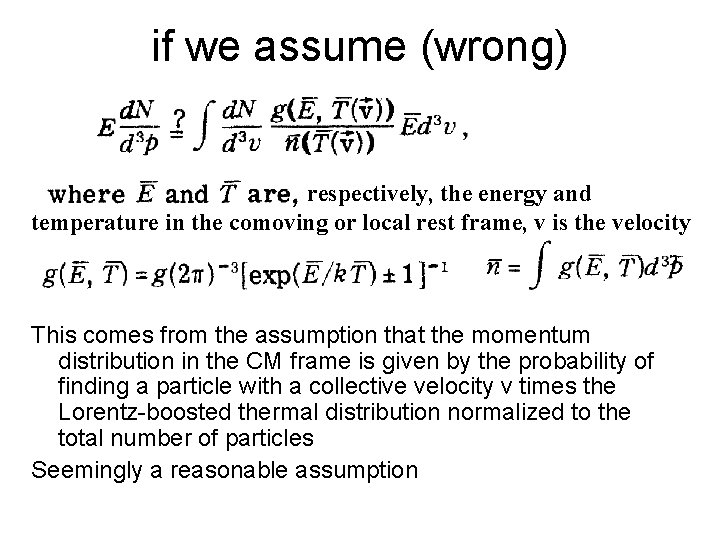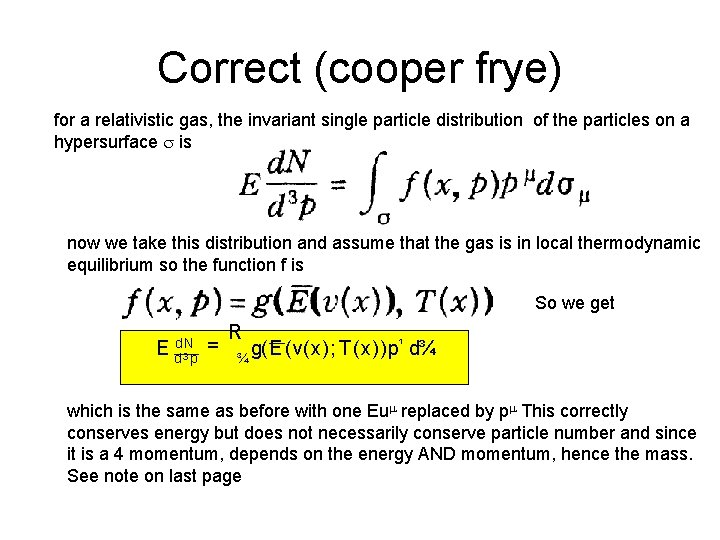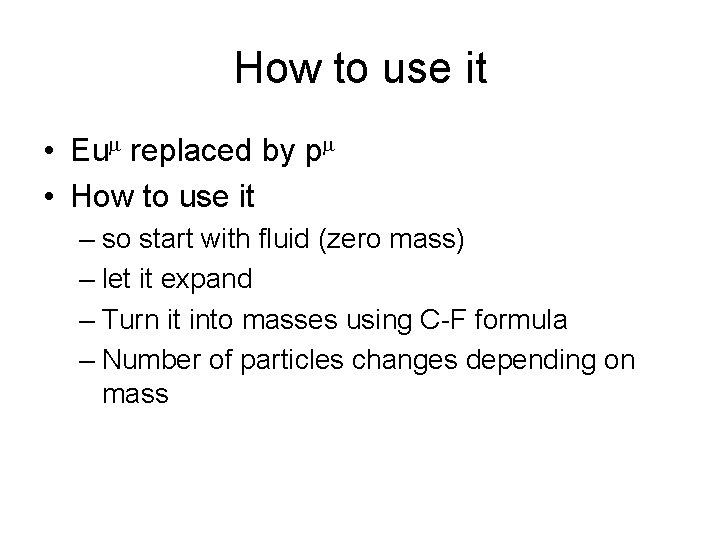# The cooperFrye formula R Seto Refs Cooper Frye

• Slides: 7The cooper-Frye formula R. Seto Refs Cooper, Frye PRD vol 10, p 186 (1974) Cooper, Frye, Shonberg, PRD vol 11 p 192 (1975)The question One statement one hears is that hydro evolves a continuous fluid (correct) and then uses the Cooper-frye formula to turn it into massive particles So, how then is the essentially fluid of essentially massless particles (quarks and gluons to make a concrete picture – but hydro doesn’t care – it could be water, or liquid helium. . ) turned into massive particles (e. g. via chiral symmetry restoration) How is the kinetic energy turned into momentum or mass. How can you conserve both momentum and energy. The misunderstanding I have had comes from the fact that I thought of particles – gaining mass – In reality I think this is what happens through a complicated set of interactions with the vacuum, as the vacuum evolves. But this is not how hydro/Cooper Frye thinks about it. Cooper frye takes this continuous liquid and then breaks it into massive particles – the number of particles formed depends on the mass. This little note will not explain WHY Cooper Frye works, but simply how it conserves energyif we assume (wrong) respectively, the energy and temperature in the comoving or local rest frame, v is the velocity This comes from the assumption that the momentum distribution in the CM frame is given by the probability of finding a particle with a collective velocity v times the Lorentz-boosted thermal distribution normalized to the total number of particles Seemingly a reasonable assumptionwe get • This eqn yields the correct number of particles but is inconsistent with energy conservation – They prove this in the paper – they figure out the total energy of the gas (fluid) and compare it to just adding up all of the momentum assuming a relativistic gas of mass m under the assumption that it is in local thermodynamic equilibrium which will be shown on the next slide – is the freezeout hypersurface – This eqn has some additional assumptions (that d. N/d 3 v entropy density) – but I think that is beside the point. One sees the same problem of energy conservationCorrect (cooper frye) for a relativistic gas, the invariant single particle distribution of the particles on a hypersurface is now we take this distribution and assume that the gas is in local thermodynamic equilibrium so the function f is So we get E d. N d 3 p = R ¾ g(E (v(x); T (x))p¹ d¾¹ which is the same as before with one Eu replaced by p This correctly conserves energy but does not necessarily conserve particle number and since it is a 4 momentum, depends on the energy AND momentum, hence the mass. See note on last pageHow to use it • Eu replaced by p • How to use it – so start with fluid (zero mass) – let it expand – Turn it into masses using C-F formula – Number of particles changes depending on massNote on number conservation from Cooper and Frye